Function Repository Resource:

# FunctionDiscontinuities

Compute the discontinuities of a function of a single variable

Contributed by: Wolfram|Alpha Math Team
 ResourceFunction["FunctionDiscontinuities"][f,x] computes the values of x at which f(x) is discontinuous with respect to x. ResourceFunction["FunctionDiscontinuities"][{f,cond},x] computes the x for which cond is True and f(x) is discontinuous with respect to x. ResourceFunction["FunctionDiscontinuities"][…,x,"Properties"] computes points of discontinuity along with related information about each.

## Details

ResourceFunction["FunctionDiscontinuities"] has the attribute HoldFirst.
ResourceFunction["FunctionDiscontinuities"] takes the option "ExcludeRemovableSingularities", having default value False, that determines whether to exclude removable discontinuities from the result.
A function f(x) is said to have a removable discontinuity at a point x=a if the limit of f(x) as xa exists and is independent of the direction in which the limit is taken, but has a value that is different from f(a) (which may or may not be defined).

## Examples

### Basic Examples (6)

Compute the points of discontinuity of a rational function:

 In:=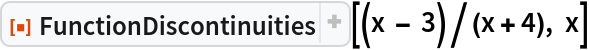Out=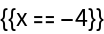Repeat the calculation, classifying the points of discontinuity:

 In:=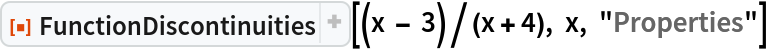Out=Compute the points of discontinuity of a trigonometric function:

 In:=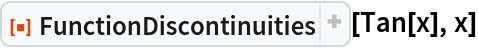Out=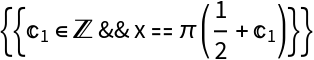Repeat the calculation, classifying the points of discontinuity:

 In:=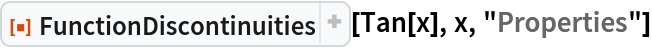Out=Compute the points of discontinuity of a smooth function:

 In:=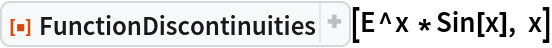Out=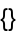Compute and plot the points of discontinuity of a rational function:

 In:=Out=In:=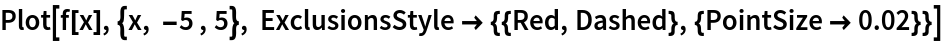Out=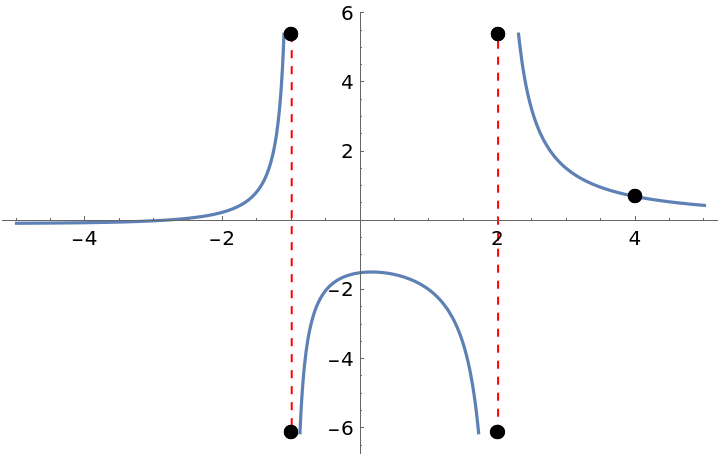Compute and plot the points of discontinuity of a step function:

 In:=Out=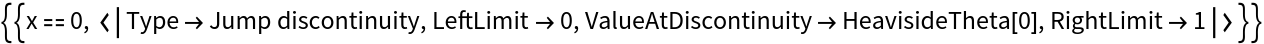In:=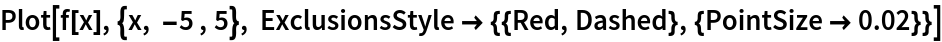Out=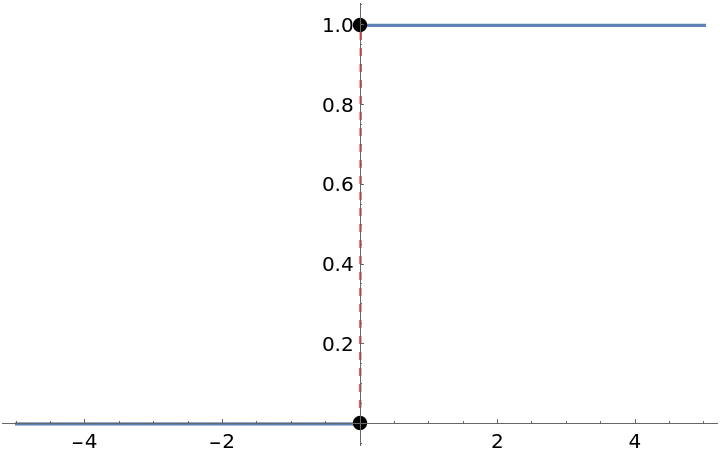Compute and plot the points of discontinuity of a step function:

 In:=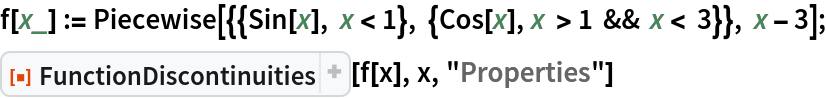Out=In:=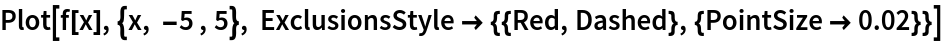Out=### Options (1)

Choose whether to exclude removable singularities:

 In:=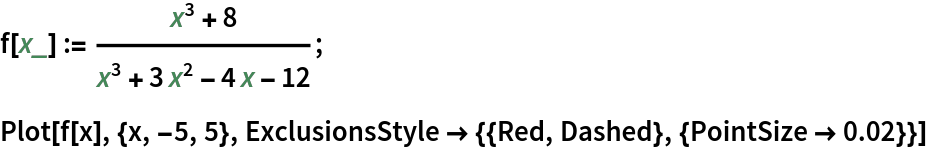Out=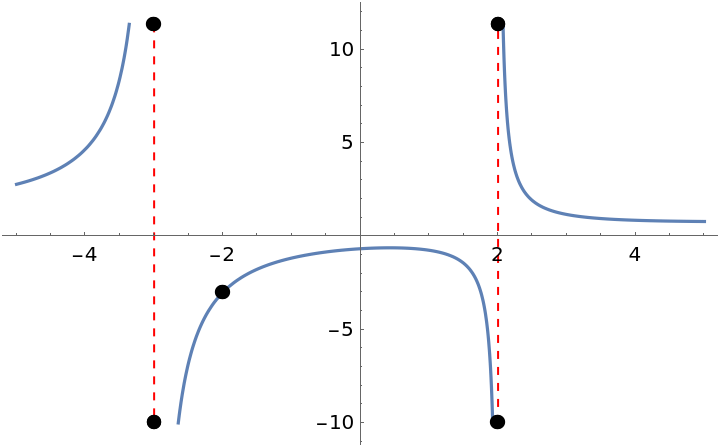In:=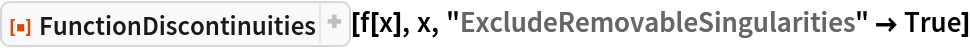Out=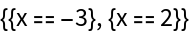In:=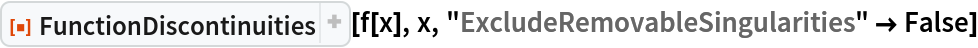Out=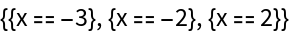### Properties and Relations (1)

FunctionDiscontinuities has the attribute HoldFirst, enabling calculations such as the following:

 In:=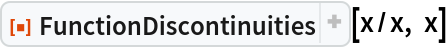Out=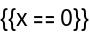### Possible Issues (1)

Points where a function approaches ± are considered to be points of discontinuity, even if they are technically outside the range of function definition:

 In:=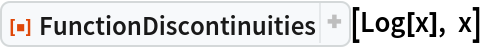Out=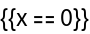## Publisher

Wolfram|Alpha Math Team

## Version History

• 10.0.1 – 27 March 2023
• 10.0.0 – 23 March 2023
• 9.0.0 – 23 March 2023
• 8.0.0 – 24 March 2020
• 7.0.0 – 10 September 2019
• 6.0.0 – 06 September 2019
• 5.0.0 – 05 September 2019
• 4.0.0 – 31 July 2019
• 3.0.0 – 12 June 2019
• 2.0.0 – 11 June 2019
• 1.0.0 – 09 January 2019